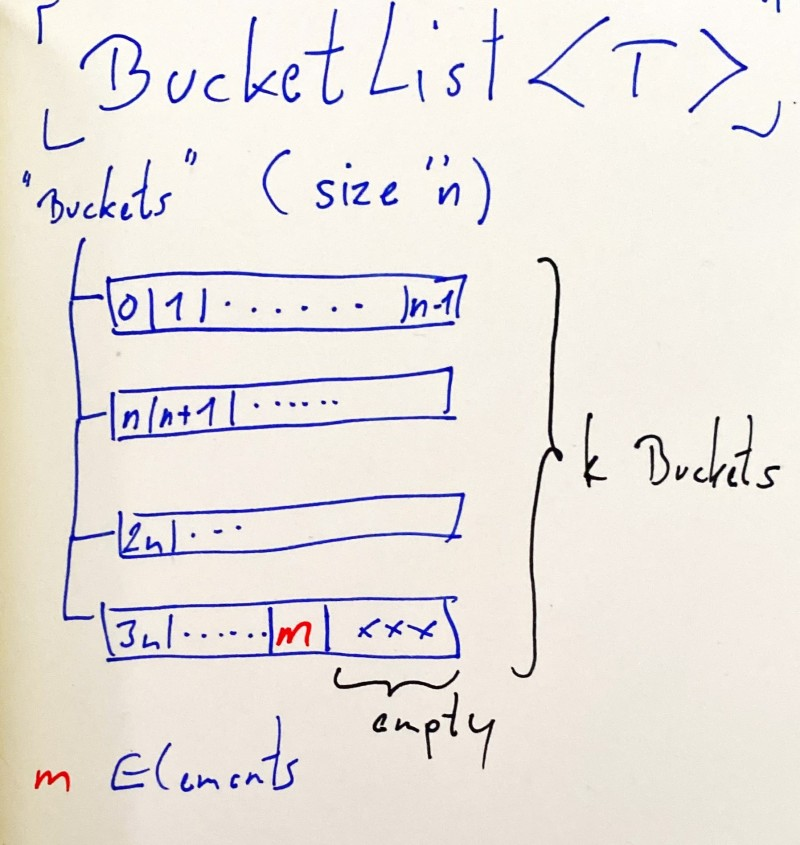# wolfgang ziegler

„make stuff and blog about it“

# BucketList<T>

March 21, 2021

TL;DR: `BucketList<T>` is a data structure that performs exceptionally well for a certain usage scenario:

• `n` (list size) is large.
• Fast index-based access: `list.GetItemAt(i)`.
• Fast index-based removal: `list.RemoveAt(i)`.

In my use case, this reduced the runtime from 7 hours to 17 seconds!

## An Interesting Problem

In December of last year I took part in solving the Advent of Code programming puzzles for the first time. Since then I became a bit obsessed with that and started solving also the older events going back until 2015.

Day 19 of 2016 presented an interesting challenge that took me a while to solve (with a reasonable runtime) and led to the solution based on this `BucketList<T>` data structure.

The basic idea behind this problem is that we have a:

• Circular data structure.
• Maintain a current element.
• Find the element opposite of the current element.
• Advance the current element by one.
• Repeat until the list has only one element left.

## A poor solution

My first approach was using a `LinkedList` which performed OK for small list sizes. But for large lists (e.g. 3.001.330 was my puzzle input) the performance was dismal.

``````List size: 3001330.
END after 26696.1142087 seconds.
``````

That's a runtime of more than 7 hours 😬.

The problem was the linear search for locating the opposite element in the list that caused an `O(n²)` complexity.

Using a simple `List<T>` (instead of `LinkedList<T>`) performed equally bad because in that case the remove operation causes a runtime complexity of `O(n²)`.

To sum it up:

Data Structure Lookup Removal
`List<T>` `O(1)` `O(N²)`
`LinkedList<T>` `O(N²)` `O(1)`

## A better (by a lot) solution

So I needed a data structure with linear or better performance for:

• Indexing
• Removal

My solution was a custom data structure I called `BucketList` (ha ha) which:

• maintains the elements in a list of lists
• that have the same maximum size (hence, buckets).This way, when performing an index-based lookup I have nearly constant¹ `O(1)` access to elements and removal is only limited by the `List<T>` performance of a single bucket.

¹ Actually `O(k)` where `k` amounts to the number of buckets which should be negligibly low compared to `n`.

To sum it up:

Data Structure Lookup Remove
`BucketList<T>` `O(k)` `O(n)`

`k`: number of buckets
`n`: bucket size (list size `N` / `k`)

This brought the runtime down dramatically!

``````List size: 3001330.
END after 17.0722168 seconds.
``````

17 seconds ... way better 🤩.

Here's the full source code for BucketList.

Note: A bucket size of `25.000` worked best in that specific scenario but your mileage may vary, of course, and depends on the problem input size.

### Caveats

• I only used `BucketList<T>` for this specific problem so far.
• The runtime performance depends on the selection of a bucket size for a given list size.
• The `InsertAt` case is not yet considered.
• I'm sure that this data structure exists already with a different name. However, I came up with this on my own when solving my problem, so give me that ;-).